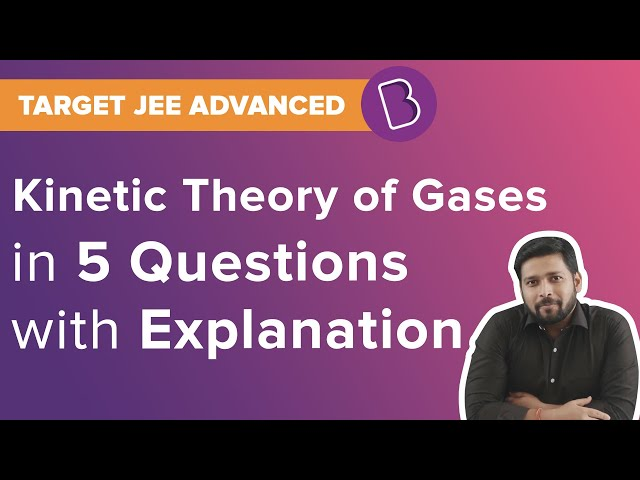# CBSE Class 11 Physics Notes Chapter 13 Kinetic Theory

## What is Kinetic Theory of Gas?

The kinetic theory of gases explains the behavior of molecules, which should further explain the behavior of an ideal gas. Ideal Gas equation consists of the pressure (P), volume (V), and temperature (T) of gases at low temperature and the equation is: PV = nRT

Where n = number of moles in the gas R = gas constant having value 8.314JK−1mol−1

Now, any gas following this equation is termed as an ideal gas. Below are some certain assumptions that we consider for describing ideal gas behavior.### What are the Assumptions of Kinetic Theory of Gases?

• All gas molecules constantly move in random directions.
• The size of molecules is very less than the separation between the molecules
• The molecules of the sample do not exert any force on the walls of the container during the collision when the gas sample is contained.
• It has a very small time interval of collision between two molecules, and between a molecule and the wall.
• Collisions between molecules and wall and even between molecules are elastic in nature.
• Newton’s laws of motion can be seen in all the molecules in a certain gas sample.
• With due course of time, a gas sample comes to a steady state. The molecule’s distribution and the density of molecules do not depend on the position, distance and time.

In-depth Reading: Kinetic Theory Of Gases

### What is law of equipartition of energy?

According to this law, when a system is in equilibrium provided the temperature is absolute, the total energy tends to be distributed evenly in the various energy modes of the absorption. Each translational and the rational degree of freedom represents one energy mode of absorption.

With the help of this law, we can determine the values of molar specific heat of gases.

### Important Questions

1. Find out the ratio of the molecular volume to actual volume occupied by the molecules of oxygen gas at STP. Let’s assume the diameter of an oxygen molecule to be 3 Å.
2. When 1 mol of any gas has a molar volume of 13.2 at standard temperature and pressure (STP: 1 atmospheric pressure, 0 °C). Show the value to be 22.4
3. At the bottom of the lake, the volume of an air bubble is 1.0 cm3 and it rises from the bottom to the top. The depth is 40 m and the temperature is 12 °C. Calculate the volume when it reaches the surface at a temperature of 35 °C?

More Questions: Important Questions – Kinetic Theory

There is a lot more to be learned about the kinetic theory of gases for Class 11. Explore more on physics formulas and physics calculators at BYJU’S

## Frequently asked Questions on CBSE Class 11 Physics Notes Chapter 13: Kinetic Theory

### What is the Ideal gas equation?

The ideal gas law (PV = nRT) relates the macroscopic properties of ideal gases.

### What does the ‘Molecular theory of gases’?

The Kinetic Molecular Theory explains the forces between molecules and the energy that they possess.

### How many gas laws are present?

Gas Laws: Boyle’s Law, Charle’s Law, Gay-Lussac’s Law, Avogadro’s Law.